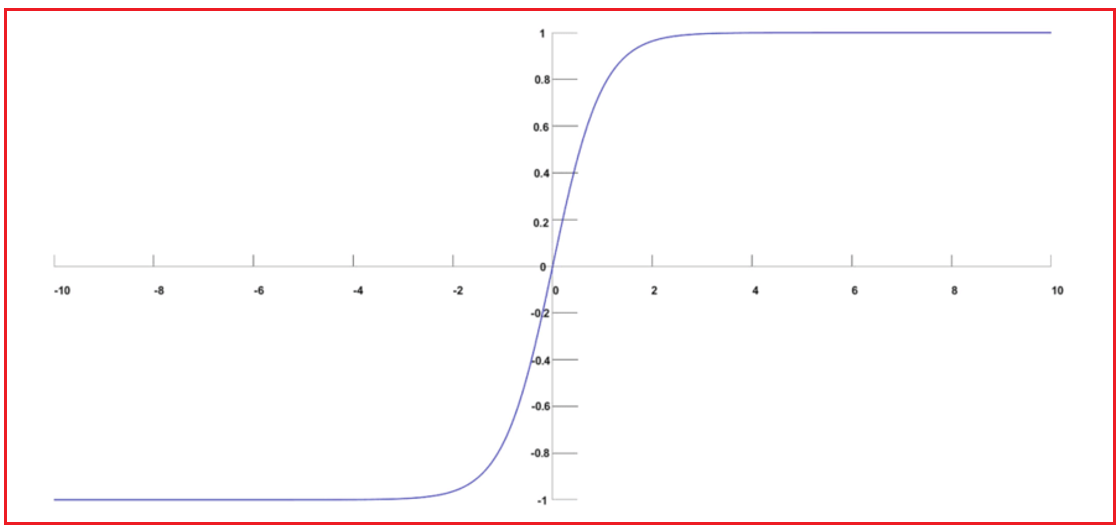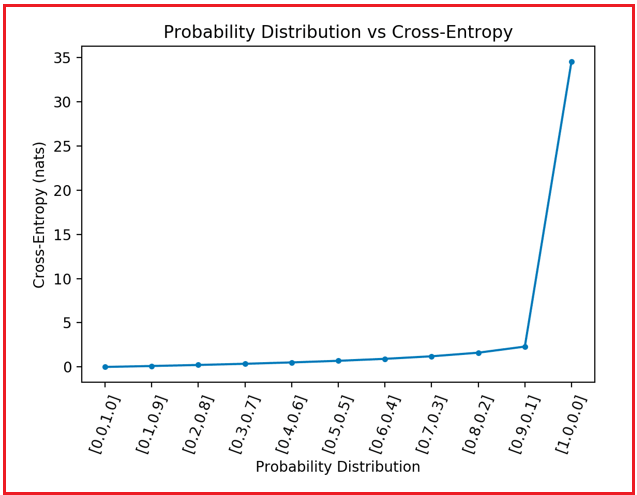# Softmax vs Cross Entropy in CNN

## SoftMax vs Cross Entropy in CNN

In this article, I am going to discuss SoftMax vs Cross Entropy in CNN. Please read our previous article where we discussed Case studies in the Image Recognition Domain.

##### SoftMax vs Cross Entropy in CNN

In classification tasks, SoftMax and cross-entropy loss are frequently employed combined, particularly in multi-class classification problems. Cross-entropy loss is a loss function, whereas SoftMax is an activation function.

The logistic function has been generalized into the SoftMax function. It accepts a vector of K real numbers and outputs a new vector of K values ranging from 0 to 1, which adds to 1. The SoftMax function’s output may be viewed as a probability distribution, with each element representing the likelihood that the input belongs to a certain class.Cross-entropy loss is a loss function that is often used in classification problems. It is determined as the negative log-likelihood of the true labels given the model’s expected probabilities. To put it another way, it assesses how well the projected probability matches the real labels.Because the output of the SoftMax function may be understood as a probability distribution across the classes, the cross-entropy loss is frequently employed in conjunction with it. The cross-entropy loss may then be used to compute the loss by comparing the anticipated probability distribution to the true distribution.

One benefit of combining SoftMax and cross-entropy loss is that they can handle several classes and output probability for each. When compared to a binary cross-entropy loss function, which can only handle two classes, this makes training a model to predict several classes easier.